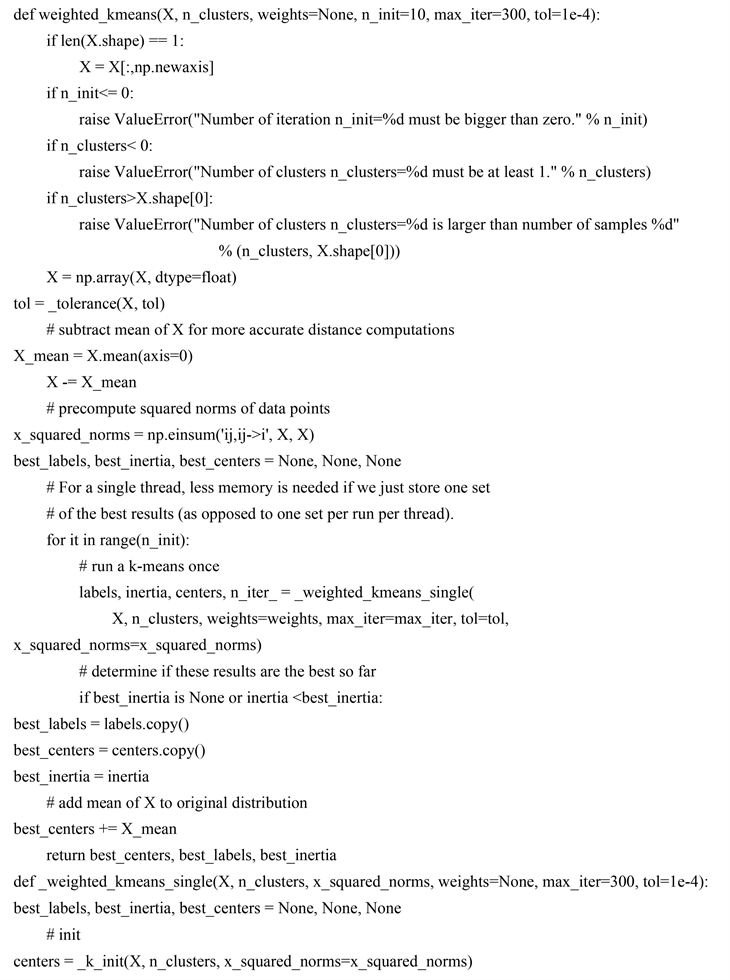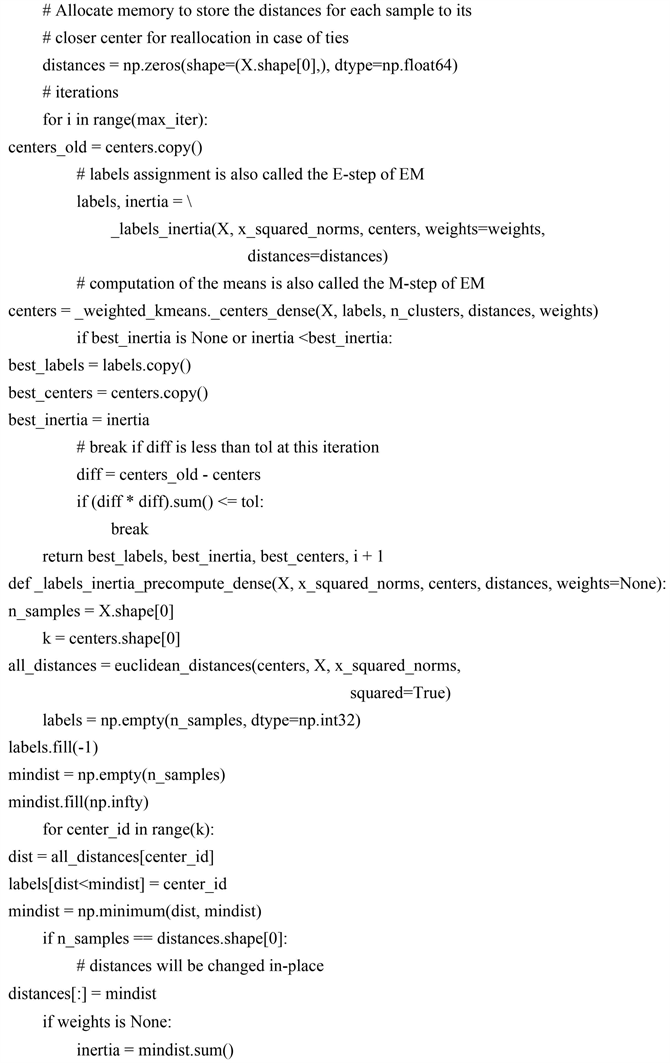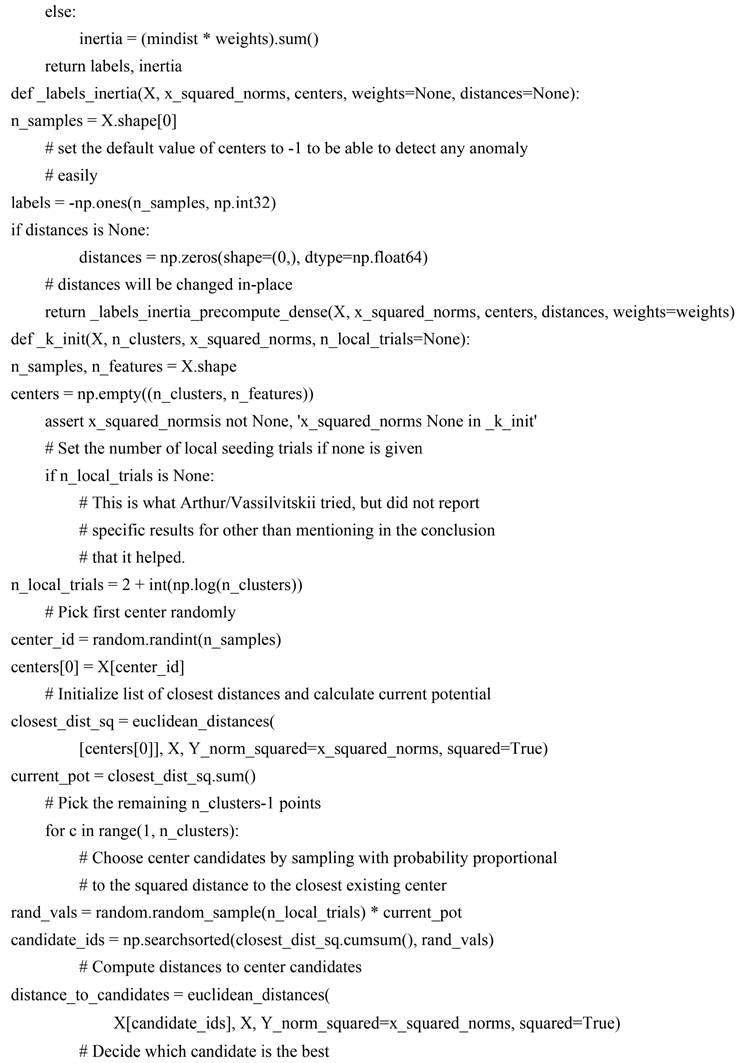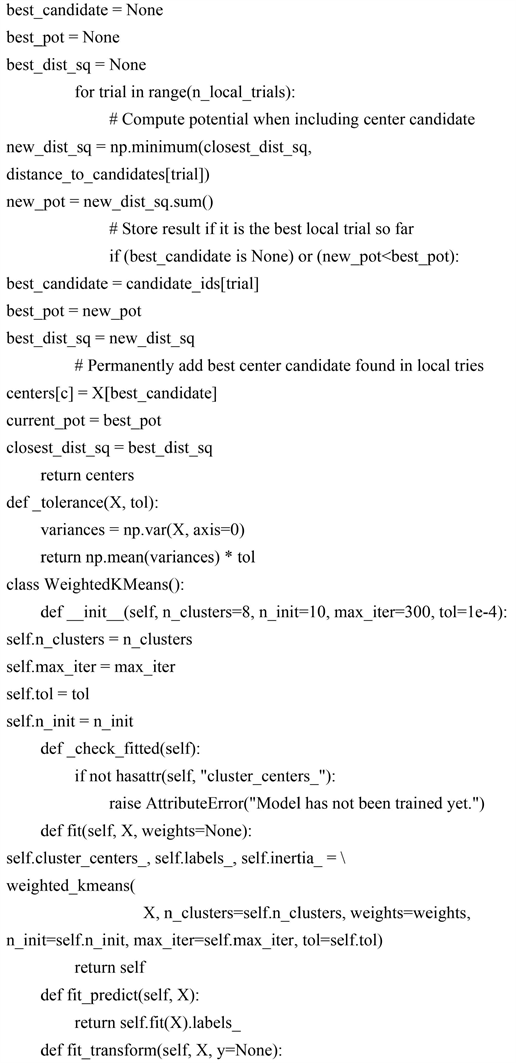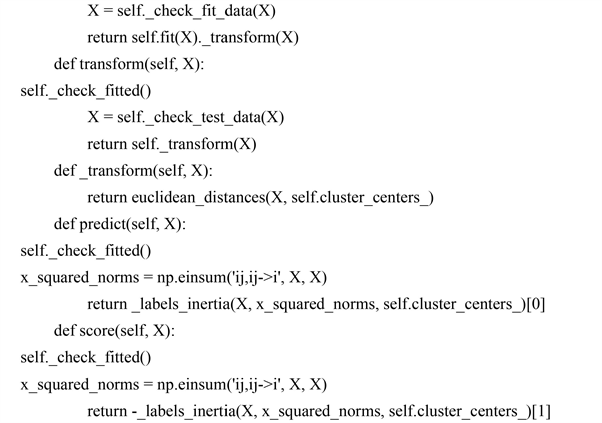﻿ 基于改进的Weighted K-Means聚类的外卖员接单区域划分问题研究 Research on the Division of the Take-Out Order Region Based on Improved Weighted K-Means Clustering

Statistics and Application
Vol. 08  No. 02 ( 2019 ), Article ID: 29532 , 15 pages
10.12677/SA.2019.82023

Research on the Division of the Take-Out Order Region Based on Improved Weighted K-Means Clustering

Yunqing Ma, Chuanxin Zhang

Shanghai University of International Business and Economics, Shanghai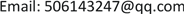Received: Mar. 6th, 2019; accepted: Mar. 21st, 2019; published: Mar. 29th, 2019ABSTRACT

The take-away industry is booming and people's demands for the quality of take-away services are constantly improving. Since the take-out area of the take-out is basically fixed, it is the key to improve efficiency by reasonably dividing the area of the take-out of the seller and assigning the number of the sellers in each area. Based on the query algorithm model, this project analyzes the take-out data of Shanghai during a certain period of time in 2017, trying to obtain a more reasonable division standard for the take-out order area and give the division. K-Means is a common partitioning clustering algorithm based on the inability of centralized system frameworks to process and analyze massive amounts of data. However, the K-Means clustering algorithm cannot be used for the two-dimensional point set with weight information. Therefore, it is especially necessary to study an improved Weighted K-Means algorithm. This project defines the weighted centroid and weighted distance, proposes a new Weighted K-Means algorithm, and uses the two methods before and after the improvement to deal with the take-out information of Shanghai take-out orders, and gives a reasonable and feasible division of the take-out area of the take-out. Comparing the results of the two methods, the improved Weighted K-Means is not only feasible, but also better in regional division. At the same time, using this method to make a new division of the take-out order area helps to optimize the existing take-away model, improve the take-out efficiency and customer satisfaction.

Keywords:Clustering Algorithm, K-Means Algorithm, Weighted K-Means Algorithm, Python1. 引言

1.1. 课题缘起

2017年，外卖行业市场规模呈现持续增长态势，交易额近千亿元人民币。随之而来的，是人们对于外卖服务质量不断提升的要求，而其中最为重要的，就是外卖送达速度。然而，商家制作外卖菜品往往时间相对固定，外卖员花在路上的时间也基本统一，因而提升外卖效率，最重要的就是提升外卖员接单效率。

1.2. 问题提出

1) 接听外卖员电话(或客户端接收外卖下单信息)；

2) 准确规范记录信息；

3) 厨房下单并加工制作；

4) 打包出品等待送餐员；

5) 送餐员取餐并送往客户指定地点；

6) 按时到达指定地点并交付；

7) 客户接到外卖，核对确认无误后结束外卖流程。

1) 被划分的区域不会重叠，没有相互之间覆盖。

2) 同一区域之间的个体相对集中，这样每一个快递员运送物资时，不会花过多时间在路程上。

3) 每一区域的外卖员数量大致相等。

1.3. 研究方法

1.4. 研究现状

1.5. 数据来源

2. 聚类模型

2.1. 聚类算法综述

2.2. K-Means算法

K-Means算法首先随机地在N个对象中选取k个数，作为初始聚类中心(即把N个对象分为k个簇)，采用距离作为相似性的评价指标，认为两个对象的距离越近，其相似度就越大。相似度通过一个簇中对象的平均值来计算。然后按照最小距离原则，讲N个对象划分到不同的簇中。最后不断迭代计算聚类中心和调整各个对象的勒边，最终使得每个对象到其判属的聚类中心的距离的平方和最小。步骤如下：

(1) 在N个对象中随机选取k个数作为初始聚类中心，即 ${c}_{1},{c}_{2},\cdots ,{c}_{k}$

(2) 将N个对象按最小距离原则找到离它最近的聚类中心 ${c}_{i}$ ，并将其划分到 ${c}_{i}$ 所标明的簇中；

(3) 计算每个簇中对象的均值，并且该均值作为该簇新的聚类中心，即

${c}_{k}=\frac{1}{n}\underset{i=1}{\overset{n}{\sum }}{X}_{i}^{\left(k\right)},k=1,2,\cdots ,K$

(4) 重复(2)~(3)步，知道没有对象或很少的对象被分配到不同的簇中。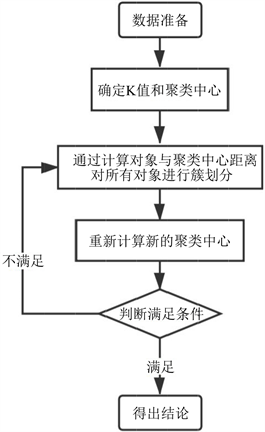Figure 1. Algorithm flowchart of K-Means

2.3. K-Means算法的Python代码

K-Means算法的Python代码如图2所示。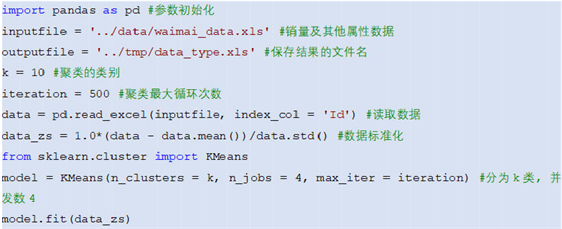Figure 2. K-Means algorithm Python code

2.4. K-Means算法的代码结果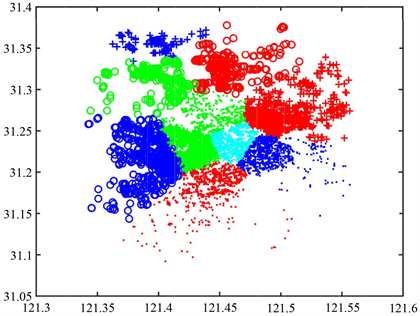Figure 3. K-Means clustering results

3. 改进的K-Means算法

3.1. 改进的基本思路

${d}_{i}=\sqrt{{\left({x}_{k}-{x}_{i}\right)}^{2}+{\left({y}_{k}-{y}_{i}\right)}^{2}}$

${d}_{i}=\frac{\sqrt{{\left({x}_{k}-{x}_{i}\right)}^{2}+{\left({y}_{k}-{y}_{i}\right)}^{2}}}{\text{weight}{\left({C}_{k}\right)}^{\gamma }}$

3.2. Weighted K-Means算法描述

${x}_{k}=\frac{\underset{i={k}_{1}}{\overset{{k}_{n}}{\sum }}{x}_{i}{w}_{i}}{\underset{i={k}_{1}}{\overset{{k}_{n}}{\sum }}{x}_{i}},{y}_{k}=\frac{\underset{i={k}_{1}}{\overset{{k}_{n}}{\sum }}{y}_{i}{w}_{i}}{\underset{i={k}_{1}}{\overset{{k}_{n}}{\sum }}{y}_{i}}$

${w}_{k}=\underset{i={k}_{1}}{\overset{{k}_{n}}{\sum }}{w}_{i}$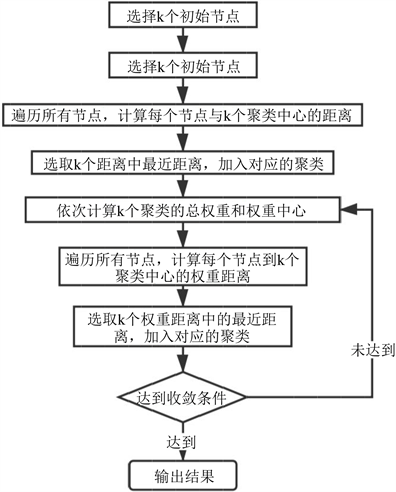Figure 4. Algorithm flowchart of Weighted K-Means

3.3. Weighted K-Means算法的Python代码

3.4. Weighted K-Means算法的代码结果

4. 结论和展望

K-Means算法作为聚类分析经典的方法之一，对大型数据集的处理效率较高，尤其是对样本分布呈现类团聚状时，可以达到很好的聚类效果。但是作为一个NP问题，它对初始聚类中心的选择以及k值的大小的确有很大的依赖性，在一定程度上限制了K-Means算法的实际应用能力。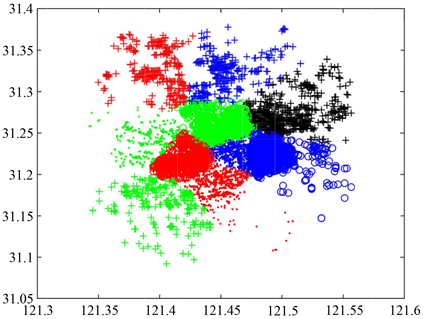Figure 5. Weighted K-Means clustering results

Research on the Division of the Take-Out Order Region Based on Improved Weighted K-Means Clustering[J]. 统计学与应用, 2019, 08(02): 203-217. https://doi.org/10.12677/SA.2019.82023

1. 1. 龚心怡, 苏燕欣, 滕明宏, 钱永贵. 当前外卖配送模式中的问题及对策分析[J]. 中国商论, 2019(3): 32-33.

2. 2. 李桃迎, 吕晓宁, 李峰, 陈燕. 考虑动态需求的外卖配送路径优化模型及算法[J]. 控制与决策, 2019, 34(2): 406-413.

3. 3. 陈霞. 计算机数据挖掘技术的开发及其应用[J]. 现代营销(经营版), 2019(2): 130-132.

4. 4. 王妍捷. 外卖O2O市场下的物流策略研究[J]. 物流技术, 2015, 34(10): 23-25.

5. 5. 黄心, 吴学群, 袁清冽. 蚁群算法在外卖配送路径规划中的应用[J]. 价值工程, 2017, 36(5): 65-67.

6. 6. 赵刚, 李昆. 几种查找算法的比较[J]. 科技信息, 2010(9): 152-168.

7. 7. 王倩影. 外卖O2O行业配送模式选择研究[D]: [硕士学位论文]. 北京: 北京交通大学, 2017.

8. 8. 饿了么星选[EB/OL]. https://star.ele.me/waimai/shop/, 2018-10.

9. 9. 李翠霞, 于剑. 一种模糊聚类算法归类的研究[J]. 北京交通大学学报: 自然科学版, 2005, 29(2): 17-21.

10. 10. 刘叶, 吴晟, 周海河, 吴兴蛟, 韩林峄. 基于K-means聚类算法优化方法的研究[J]. 信息技术, 2019, 43(1): 66-70.